### Home > MC2 > Chapter 9 > Lesson 9.3.3 > Problem9-107

9-107.
1. A triangle is enlarged by a scale factor of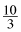. Homework Help ✎

1. If the perimeter of the enlargement is 36 m, find the perimeter of the original triangle.

2. If the area of the enlarged triangle is 25 square meters, what is the area of the original triangle?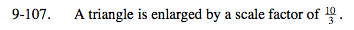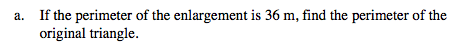According to the Math Notes box from Lesson 9.2.2: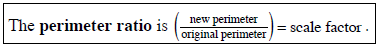$\text{What original perimeter times the scale factor }\frac{10}{3} \text{ equals the enlarged perimeter of 36 m?}$

This can be represented by the following equation with the original perimeter defined as x.

$\frac{10}{3} x = 36$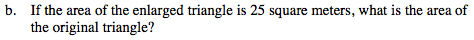According to the Math Notes box from Lesson 9.2.2: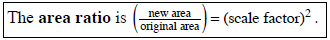$\text{What would the area be if the scale factor is } \frac{10}{3} ?$

$\text{If the area ratio is } \frac{100}{9} \text{, create an expression like the one given above to solve for the original area.}$

2.25 square meters